# SAT II Math II : Properties and Identities

## Example Questions

### Example Question #1 : Properties And Identities

What property of arithmetic is demonstrated below?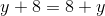Transitive

Distributive

Identity

Commutative

Associative

Commutative

Explanation:

The statement shows that two numbers can be added in either order to achieve the same result. This is the commutative property of addition.

### Example Question #2 : Properties And Identities

What property of arithmetic is demonstrated below?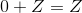Reflexive

Transitive

Inverse

Distributive

Identity

Identity

Explanation:

The fact that 0 can be added to any number to yield the latter number as the sum is the identity property of addition.

### Example Question #3 : Properties And Identities

What property of arithmetic is demonstrated below?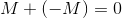Associative

Inverse

Symmetric

Transitive

Identity

Inverse

Explanation:

For every real number, there is a number that can be added to it to yield the sum 0. This is the inverse property of addition.

### Example Question #4 : Properties And Identities

What property of arithmetic is demonstrated below?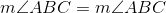Symmetric

Symmetric

Transitive

Commutative

Reflexive

Reflexive

Explanation:

That any number is equal to itself is the reflexive property of equality.

### Example Question #5 : Properties And Identities

What property of arithmetic is demonstrated below?

If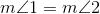then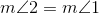.

Transitive

Commutative

Associative

Reflexive

Symmetric

Symmetric

Explanation:

If an equality is true, then it can be correctly stated with the expressions in either order with equal validity. This is the symmetric property of equality.

### Example Question #6 : Properties And Identities

What property of arithmetic is demonstrated below?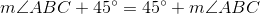Commutative

Distributive

Transitive

Identity

Associative

Commutative

Explanation:

The statement shows that two numbers can be added in either order to yield the same sum. This is the commutative property of addition.

### Example Question #7 : Properties And Identities

Which of the following sets is not closed under multiplication?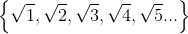All of the sets in the other four responses are closed under multiplication.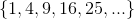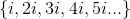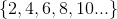Explanation:

A set is closed under multiplication if and only the product of any two (not necessarily distinct) elements of that set is itself an element of that set.is closed under multiplication since the product of two even numbers is even: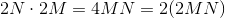is closed under multiplication since the product of two perfect squares is a perfect square: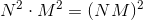is closed under multiplication since the product of two square roots of positive integers is the square root of a positve integer: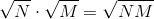But, as can be seen here, is not closed under multiplication: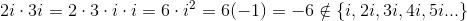### Example Question #8 : Properties And Identities

Which expression is not equal to 0 for all positive values of?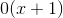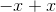All four expressions given in the other choices are equal to 0 for all positive values of.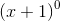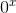Explanation:is the correct choice.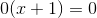for all values of, since, by the zero property of multiplication, any number multiplied by 0 yields product 0.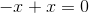for all values of- this is a direct statement of the inverse property of addition.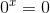, since 0 raised to any positive power yields a result of 0.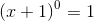, since any nonxero number raised to the power of 0 yields a result of 1.

### All SAT II Math II Resources